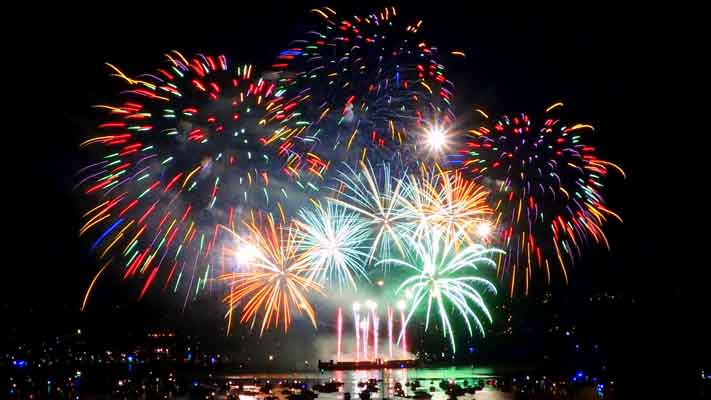(Not Double Science - Chemistry GCSE Only)

1.Sodium hydroxide was added to a pale green solution and a green precipitate formed.

Which ion was present in the pale green solution?

• A.   iron(II) ion, Fe2+
• B.   iron(III) ion, Fe3+
• C.   copper(II) ion, Cu2+
• D.   calcium ion, Ca2+
2. Fireworks have coloured flames due to the presence of metal ions.A green flame is produced by the presence of ..

• A.  calcium ions
• B.  copper(II) ions
• C.  potassium ions
• D.  lithium ions
3.A student tested a solution for the presence of iodide ions. The test was positive.

 Substances added Observation made A Nitric acid and silver nitrate White precipitate B Nitric acid and silver nitrate Yellow precipitate C Hydrochloric acid and silver nitrate White precipitate D Hydrochloric acid and silver nitrate Yellow precipitate

4+5: A white solid X was tested and gave the following results:

 Test Result Flame test orange-red flame Dilute hydrochloric acid added Bubbles seen

4. Which cation (positive ion) is present in solid X?
• A.   magnesium
• B.   calcium
• C.   lithium
• D.   sodium
5. Which anion (negative ion) is present in solid X?
• A.   chloride
• B.   sulfate
• C.   nitrate
• D.   carbonate

Q6-7:
A yellow solution Y was tested and gave the following results:

 Test Result Add sodium hydroxide solution brown precipitate Add dilute hydrochloric acid then barium chloride solution white precipitate

6. The name of the brown precipitate formed is..
• A.   iron(II)hydroxide
• B.   iron(III)hydroxide
• C.   iron(II)sulfate
• D.   iron(III)sulfate
7. The formula of the white precipitate formed is..
• A.   FeCl2
• B.   BaCO3
• C.   BaSO4
• D.   BaCl2

8. A few drops of sodium hydroxide solution were added to a colourless solution and a white precipitate was seen to form.

The precipitate dissolved when excess sodium hydroxide solution was added.
Identify the metal ion present in the solution.

• A.   calcium ion, Ca2+
• B.   magnesium ion, Mg2+
• C.   potassium ion, K+
• D.   aluminium ion, Al3+

9. A student is given an unknown solution. Which two of the following tests would provide evidence that the solution is copper(II)sulfate?

 1. Adding hydrochloric acid 2. Adding sodium hydroxide solution 3. Adding dilute nitric acid then silver nitrate 4. Adding dilute hydrochloric acid then barium chloride

• A.   1 and 3
• B.   1 and 4
• C.   2 and 3
• D.   2 and 4
10. What colour of flame would be produced by sodium nitrate?
• A.   lilac
• B.   crimson
• C.   orange-red
• D.   yellow